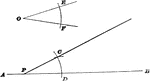### Construction Of Angle On Straight Line

Illustration used to show how to "draw a straight line through any given point on a given straight line…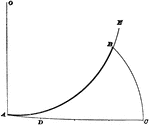### Construction Of Arc

Illustration used to show how to "find an arc of a circle having a known radius, which shall be equal…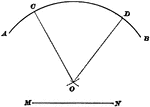### Construction Of Center

Illustration used to show how to find the center when given an arc and its radius.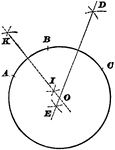### Construction Of Circumference

Illustration used to show how to pass a circumference through any three points not in the same straight…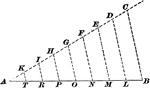### Construction Of Dividing A Line

Illustration used to show how to divide a given straight line into required number of equal parts.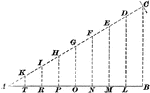### Construction Of Dividing A Line

Illustration used to show how to divide a given straight line into required number of equal parts.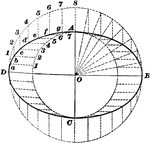### Construction Of Ellipse

Illustration used to show how to draw an ellipse when given the diameters.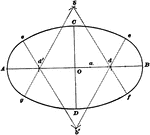### Construction Of Ellipse

Illustration used to show how to draw an ellipse by circular arcs.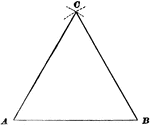### Construction Of Equilateral Triangle

Illustration used to show how to draw an equilateral triangle when given one side.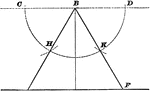### Construction Of Equilateral Triangle

Illustration used to show how to draw an equilateral triangle when given the altitude.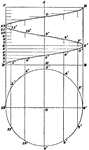### Construction Of Helix

Illustration used to show how to draw a helix when the pitch and the diameter are given.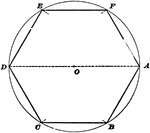### Construction Of Hexagon Inscribed In Circle

Illustration used to show how to inscribe a regular hexagon in a given circle.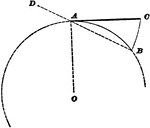### Construction Of Line Equal To Arc

Illustration used to show how to find a straight line of the same length as a given arc of a circle.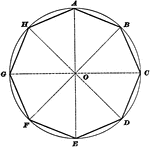### Construction Of Octagon Inscribed In Circle

Illustration used to show how to inscribe a regular octagon in a given circle.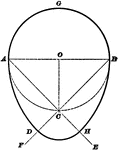### Construction Of Oval

Illustration used to show how to draw an egg-shaped oval when given the diameters.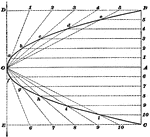### Construction Of Parabola

Illustration used to show how to draw a parabola when the axis and longest double ordinate is given.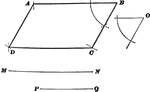### Construction Of Parallelogram

Illustration used to show how to draw a parallelogram when given the sides and one of the angles.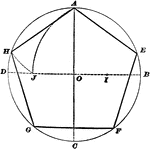### Construction Of Pentagon Inscribed In Circle

Illustration used to show how to inscribe a regular pentagon in a given circle.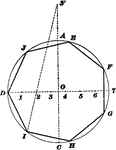### Construction Of Polygon Inscribed In Circle

Illustration used to show how to inscribe any regular polygon in a given circle.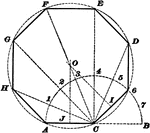### Construction Of Regular Polygon

Illustration used to show how to draw a regular polygon when given a side of the polygon.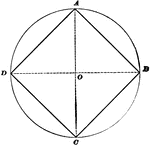### Construction Of Square Inscribed In Circle

Illustration used to show how to inscribe a square in a given circle.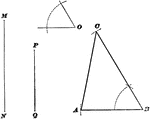### Construction Of Triangle

Illustration used to show how to draw a triangle when given two sides and the included angle of the…